Monday , May 29 2023

# Table Partitioning in PostgreSQL 11.2

We will look at the answers for the questions;

• What is partition in PostgreSQL
• What are the advantages of Table Partitioning in PostgreSQL
• PostgreSQL Partition Types

## What is Partition in PostgreSQL?

We will be discussing the table partitioning in PostgreSQL 11.2. The partitioning method used before PostgreSQL 10 was very manual and problematic. Imagine that before version 10, Trigger was used to transfer data to the corresponding partition. Imagine how old it is.

Partitioning the table according to certain criteria is called partitioning. The main table we partitioned is called master and each partition are called child.

## What are the advantages of Table Partitioning in PostgreSQL?

• Improves query performance. (Since the queries read the data only from the relevant partition, query result will be faster.)
• Index cost and Size are decreasing. We reduce the size of our indexes and decrease the index fragmentation by creating an index in the relevant partition only.
• We will be able to manage our Bulk operations healthier and faster.

## Partition Types in PostgreSQL

You can find the partition types in postgresql below.

### LIST PARTITION in PostgreSQL

The table is partitioned according to the key value of the partition column. For Example, suppose that you have a table that contains person name and country information and you want to create a partition according to the country column’s value. You can perform this operation by using LIST PARTITION.

#### PostgreSQL List Partition Example

First execute the command `\x` for user friendly screen.

Then check partitions created successfully; Write your table name instead of person in the below script if your table name is different.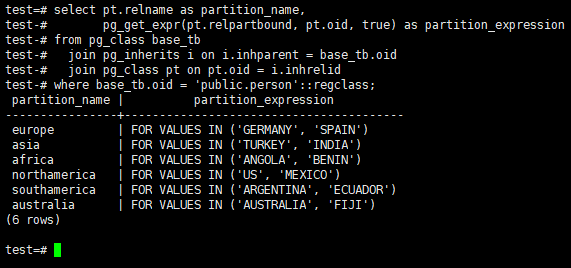Insert new records to ASIA partition. The following data will be inserted to ASIA partition. Because the values TURKEY and INDIA is in the ASIA partition.

##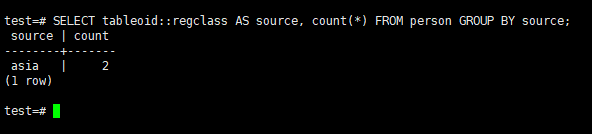Then insert new records to other partitions to see the distribution.

## RANGE PARTITION in PostgreSQL

Table partitioning is performed according to a range according to the specified criteria. For example, we can create a range partition according to a specific date range, or we can create a range partition using a range according to other data types.

#### PostgreSQL Range Partition Example

Let’s create our demo table at first.

We have specified partition type and partition column above. PARTITION BY RANGE (sales_date)

Now let’s create our Partitions. Since we will create partitions monthly, we divide our table into 12 for the last 1 year.

#### Check Partitions in PostgreSQL

After creating our partitions, let’s have a chek without inserting data.

We can check the partitions we created with the help of the below script.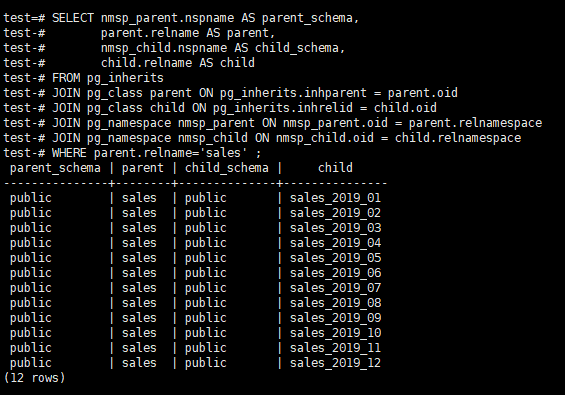After completing our checks, let’s insert data to our table.

Now let’s execute a query and check if our query brings data from the relevant partition.

Now let’s check which partitions it use with EXPLAIN.

When you execute the query, we see that it uses the sales_2019_04 and sales_2019_05 partitions.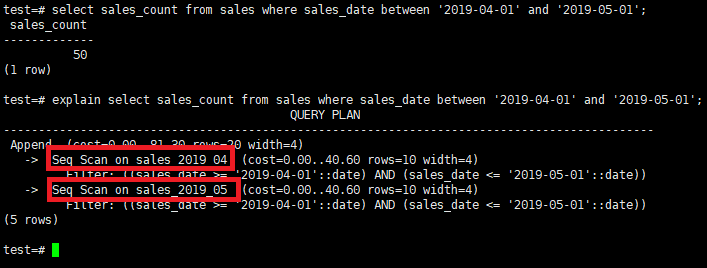#### Get Row Count in Each Partition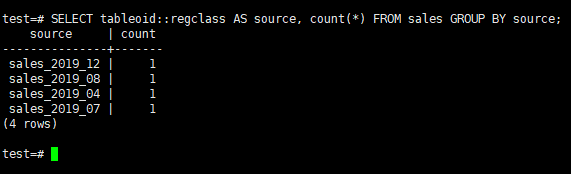## Hash PARTITION in PostgreSQL

In Hash Partition, data is transferred to partition tables according to the hash value of Partition Key(column you specified in PARTITION BY HASH statement). You can specify a single column or multiple columns when specifying the Partition Key. In order to distribute the data equally to partitions, you should take care that partition key is close to unique. It is created similar to the RANGE and LIST partition.

There are MODULUS and REMAINDER concepts during the creation of partitions tables. The MODULUS value indicates how many partition tables we have. It is fixed for all partition tables and does not change.

Since there are 10 partitions, REMAINDER can have a value from 0 to 9. If you do not specify the modulus and remainder values correctly, you will receive the below error.

ERROR: every hash partition modulus must be a factor of the next larger modulus

The hash value of the partition key used for the HASH partition is divided into MODULUS value and the data is transferred to the REMAINDER table pointed to by the remaining value.

For Example, suppose that the hash value is 102. It divides 102 by 10. Here, the remaining value is 2. So, the data will go to the REMANDER 2 table.

#### PostgreSQL Hash Partition Example

In this example, we will use the same table structure as the List Partition Example. But the partition column will be PersonName. But do not use name column as hash partition column in your production environment. Because names are often not unique. Therefore, data is not evenly distributed across partitions.

Note: Do not forget person table we have created for previous example.

You can check partition is created with the command `\d+ person`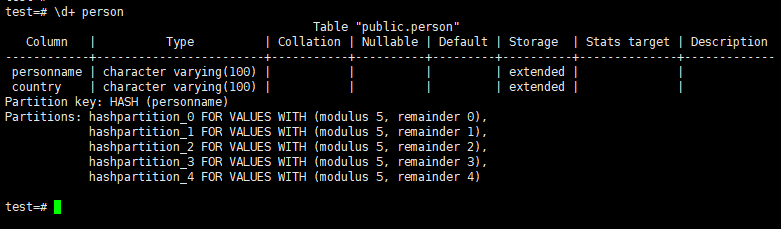Insert Into data to the table. Note that we insert 3 row and the names of the 2 rows are the same. This will cause the data not to be evenly distributed across partition tables.

Select * from the main table and partition tables as below. You will see that there are no rows in the main table. Two rows will be on a partition because of two rows name value is the same and the other row will be in different partition.

If you select maint table without only, you can see all the rows;

You can see the distribution with the below query;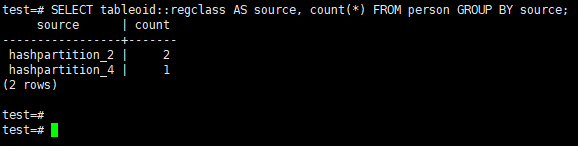## Sub-Partitioning in PostgreSQL

With Sub Partition, we can divide the partitions of the tables into sub-partitions. For example, suppose you have a partitioned table by years. It means a partition for each year. But you may also want to make partitions by months.

#### PostgreSQL Sub Partition Example

In this example, we will use the same table structure as the Range Partition Example.

Note: Do not forget sales table we have created for previous example.

Create partition for 2018-2019-2020.

Insert below records to table.

#### Get Row Count in Each Partition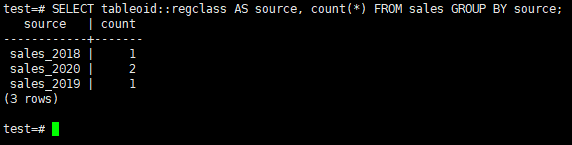Suppose that your needs has changed and you need also sub partitions for new year. To perform this we will create a partition for sales_2021, and subpartitions for each month in 2021.

The last partition structure of our table is as follows.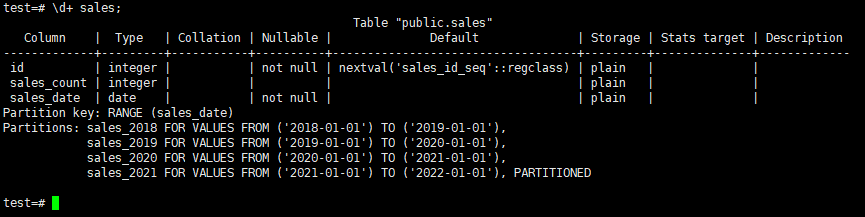if you want to see the sub partitions you should execute the `\d+ sales_2021` command.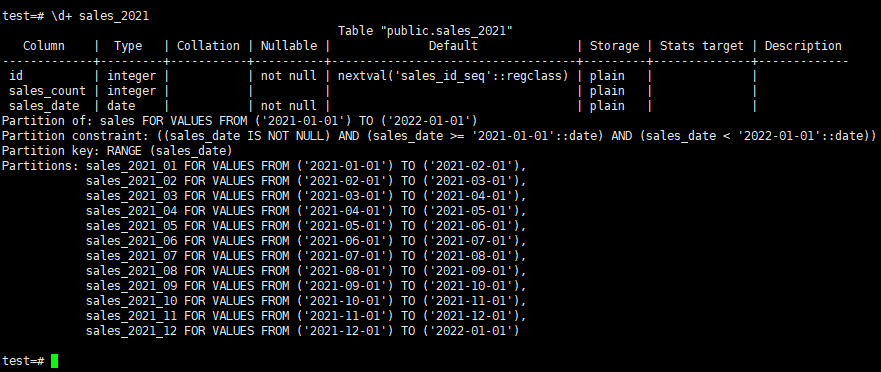1.